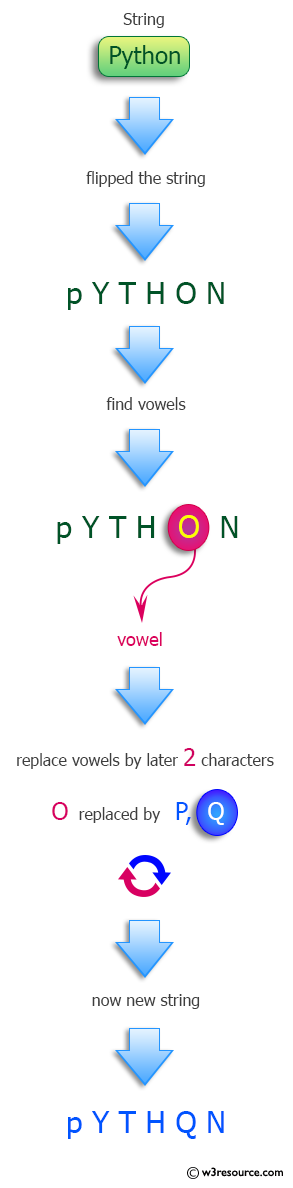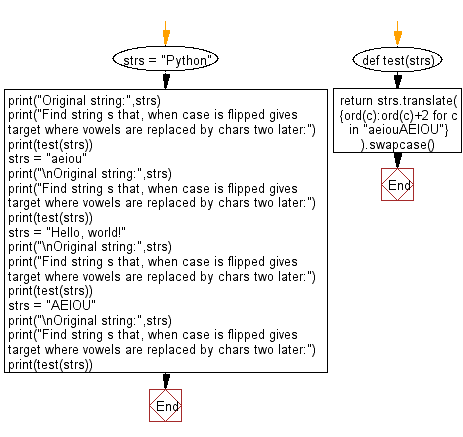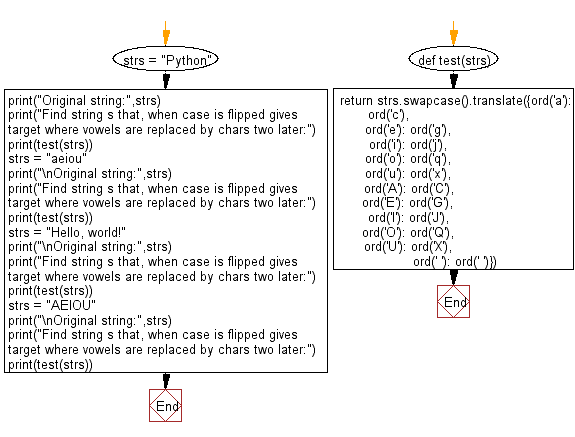﻿ Python: Find string s that, when case is flipped gives target where vowels are replaced by chars two later - w3resource# Python: Find string s that, when case is flipped gives target where vowels are replaced by chars two later

## Python Programming Puzzles: Exercise-40 with Solution

Write a Python program to find string s that, when case is flipped gives target where vowels are replaced by chars two later.

```Input: Python
Output:
pYTHQN

Input: aeiou
Output:
CGKQW

Input:  Hello, world!
Output:
hGLLQ, WQRLD!

Input: AEIOU
Output:
cgkqw
```

Pictorial Presentation:Sample Solution-1:

Python Code:

``````#License: https://bit.ly/3oLErEI

def test(strs):
return strs.translate({ord(c):ord(c)+2 for c in "aeiouAEIOU"}).swapcase()

strs = "Python"
print("Original string:",strs)
print("Find string s that, when case is flipped gives target where vowels are replaced by chars two later:")
print(test(strs))
strs = "aeiou"
print("\nOriginal string:",strs)
print("Find string s that, when case is flipped gives target where vowels are replaced by chars two later:")
print(test(strs))
strs = "Hello, world!"
print("\nOriginal string:",strs)
print("Find string s that, when case is flipped gives target where vowels are replaced by chars two later:")
print(test(strs))
strs = "AEIOU"
print("\nOriginal string:",strs)
print("Find string s that, when case is flipped gives target where vowels are replaced by chars two later:")
print(test(strs))
``````

Sample Output:

```Original string: Python
Find string s that, when case is flipped gives target where vowels are replaced by chars two later:
pYTHQN

Original string: aeiou
Find string s that, when case is flipped gives target where vowels are replaced by chars two later:
CGKQW

Original string: Hello, world!
Find string s that, when case is flipped gives target where vowels are replaced by chars two later:
hGLLQ, WQRLD!

Original string: AEIOU
Find string s that, when case is flipped gives target where vowels are replaced by chars two later:
cgkqw
```

Flowchart:## Visualize Python code execution:

The following tool visualize what the computer is doing step-by-step as it executes the said program:

Sample Solution-2:

Python Code:

``````#License: https://bit.ly/3oLErEI

def test(strs):
return strs.swapcase().translate({ord('a'): ord('c'),
ord('e'): ord('g'),
ord('i'): ord('j'),
ord('o'): ord('q'),
ord('u'): ord('x'),
ord('A'): ord('C'),
ord('E'): ord('G'),
ord('I'): ord('J'),
ord('O'): ord('Q'),
ord('U'): ord('X'),
ord(' '): ord(' ')})

strs = "Python"
print("Original string:",strs)
print("Find string s that, when case is flipped gives target where vowels are replaced by chars two later:")
print(test(strs))
strs = "aeiou"
print("\nOriginal string:",strs)
print("Find string s that, when case is flipped gives target where vowels are replaced by chars two later:")
print(test(strs))
strs = "Hello, world!"
print("\nOriginal string:",strs)
print("Find string s that, when case is flipped gives target where vowels are replaced by chars two later:")
print(test(strs))
strs = "AEIOU"
print("\nOriginal string:",strs)
print("Find string s that, when case is flipped gives target where vowels are replaced by chars two later:")
print(test(strs))
``````

Sample Output:

```Original string: Python
Find string s that, when case is flipped gives target where vowels are replaced by chars two later:
pYTHQN

Original string: aeiou
Find string s that, when case is flipped gives target where vowels are replaced by chars two later:
CGJQX

Original string: Hello, world!
Find string s that, when case is flipped gives target where vowels are replaced by chars two later:
hGLLQ, WQRLD!

Original string: AEIOU
Find string s that, when case is flipped gives target where vowels are replaced by chars two later:
cgjqx
```

Flowchart:## Visualize Python code execution:

The following tool visualize what the computer is doing step-by-step as it executes the said program:

Python Code Editor :

Have another way to solve this solution? Contribute your code (and comments) through Disqus.

What is the difficulty level of this exercise?

Test your Programming skills with w3resource's quiz.

﻿

## Python: Tips of the Day

Clamps num within the inclusive range specified by the boundary values x and y:

Example:

```def tips_clamp_num(num,x,y):
return max(min(num, max(x, y)), min(x, y))
print(tips_clamp_num(2, 4, 6))
print(tips_clamp_num(1, -1, -6))
```

Output:

```4
-1
```